# Running Total Expression for RDLC

Overview:

running total is the addition of a sequence of numbers that is updated each time a new number is added to the sequence. We can calculate the running total by adding the value of the new number to the previous running total. It helps us calculate the total at any point without having to sum the entire sequence each time.

Scenario:

Create a report in RDLC format: From the customer ledger entry, for each customer, calculate the remaining balance as per document number in running total format.

Solution:

• Create a report object, define the data item as a “Cust. ledger entry,” define the required fields, and build a report.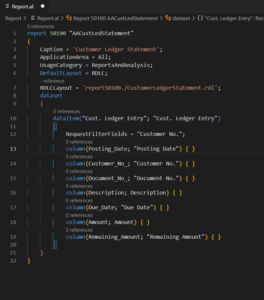• After building the report, start designing a report as per the requirements.

Now, I want to calculate the running total of the remaining amount in the last column, as highlighted below. Simply right-click on the data field of the remaining amount and click on the expression.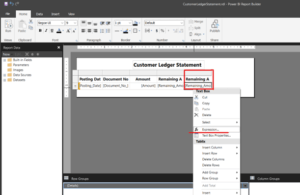• As highlighted, we can see that in aggregate functions, there is an expression for the running value. First, use the expressions given in the below format: define the field that you want to calculate in running value format; then, at last, define your initial group name, like customer_No_.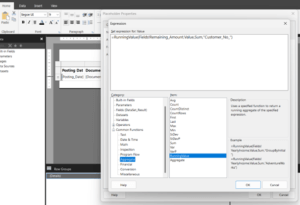• We can find the initial group name by clicking on group properties, as shown below.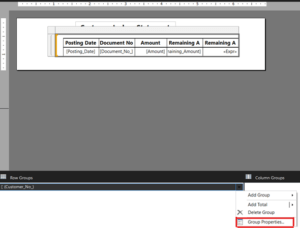• After saving the report, you can find the calculation of the remaining amount in running value format as given below.

Output: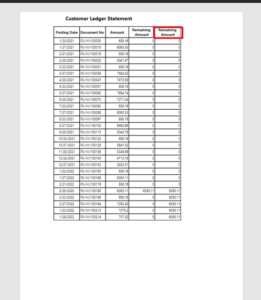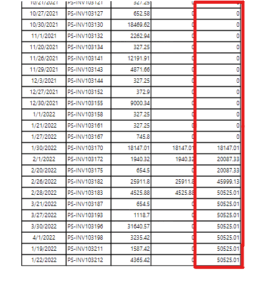Thank you!

Samiksha S. Mhatre